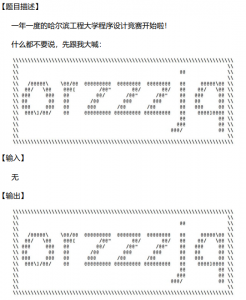• 2019-03-09
• 1,292
• 0
• 0

# C++11新特性

## 1. 同步互斥锁及多线程

（后续补充）

• std::lock_guard
• std::mutex
• std::atomic
• std::chrono

## 2. STL容器中emplace函数

emplace与insert的不同在于：

• emplace是构造新对象，参数会传递给元素的构造函数emplace函数的参数根据元素类型而变化，参数必须与元素类型的构造函数相匹配
• insert/push是拷贝对象，把对象拷贝到容器中。

（据说）emplace比insert在速度上有了很大的提升。

## 3. auto关键字

##### example 1:
``````auto i = 3.5
``````

``````double i = 3.5
``````

##### example 2:
``````std::set<int> st;
for (auto item : st) {
std::cout << item << std::endl;
}
``````

``````std::set<int> st;
std::set<int>::iterator it;
for (it = st.begin(); it != st.end(); it++) {
std::cout << *it << std::endl;
}
``````

## 4. 原生字符串C++11中的原生字符串功能就显得非常便捷。

``````old_str = "C:\\Program Files\\Microsoft.NET\\ADOMD.NET";

``````str = R"(this is the string)";
str = R"other(this is the string)other";``````

# C++基础知识、注意事项

### 1. 在C++中不建议使用宏，应尽可能地使用const常量或inline内联函数代替

##### example 1.
``#define PI 3.14    ==>    const double PI = 3.14``

##### example 2.
``#define SQR(x) x*x``

##### example 3.
``#define SQR(x) ((x)*(x))``

### 2. C/C++宏中#和##的用途

``````#define a(x) tree##x
#define b(x) #x

/* ------------- */

int tree1 = 10;
cout << a(1) << endl;     // cout << tree1 << endl;
cout << b(test) << endl;  // cout << "test" << endl;``````